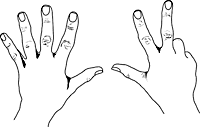# Primary One Preparation – Mathematics (Part 2)(Updated 31 Dec 2008) Now that you know what your child will be required to do in Primary One for Mathematics, the question is how can you help prepare your child so that he/she can cope with the subject.

With proper guidance, it should not be too difficult for the average child to undergo the following progress schedule.  Please note that this should NOT be taken as a definitive guide to gauge the ability of children!

• 1-2 years old
• Recognize and say the 10 numeral digits from 0 – 9 in the correct sequence
• Recognize shapes (circle, triangle, rectangle, square)
• Count objects up to 10
• 3-4 years old
• Write the 10 numeral digits
• Finger math (show 0 to 9 using fingers)
• Count objects up to 100
• Count down from 20
• Differentiate between ordinal, cardinal and nominal numbers
• Ordinal: The number is used to indicate the order of things in a set, ie. 1 comes before 2, 3 comes after 2.  They show the rank or position, eg. First (1st), Second (2nd), Third (3rd), etc.
• Cardinal: The number is used to indicate quantity.  It answers the question of “how many?”.  This is normally the result of counting, eg. 3 apples, 10 rabbits, 1 me.
• Ordinal: The number is used as an identity.  Eg.  My home block number is 27.  My birthday is on 5 Feb.  My telephone number is 67654321.  My favorite bus is No. 197.
• Compare:
• Quantity: More, equal, less
• Size: Bigger, same size, smaller
• Length: Taller (longer), same height (length), shorter
• Weight: Heavier, same weight, lighter
• Speed: Faster, same speed, slower
• Time:
• 7 o’clock in the Morning, 12 o’clock in the Afternoon, 7 o’clock at Night
• 5-6 years old :
• Perform simple addition and subtraction of totals up to 10
• 10 = 1+9 = 2+8 = 3+7 = 4+6 = 5+5 = 6+4 = 7+3 = 8+2 = 9+1
• 9 = 1+8 = 2+7 = 3+6 = 4+5 = 5+4 = 6+3 = 7+2 = 8+1
• 8 = 1+7 = 2+6 = 3+5 = 4+4 = 5+3 = 6+2 = 7+1
• Simple number series
• eg. 1, 3, 5, 7, … (odd numbers)
• eg. 5, 10, 15, 20, … (5’s)
• Up to 5 times table in terms of groups of 1’s, 2’s, 3’s, 4’s and 5’s.
• Recognise and write the English equivalents of the numerals
• Read the 12 hours of the analog clock, and apply AM and PM
• Identify Singapore coins and notes of different denominations, up to S\$10.
• Tell the value of money by summing up coins, up to S\$1. (No decimal point)
• Identify 3D shapes: cube, box, cone, sphere, cylinder and the number of 2D faces they present

In general, if the child possesses the above skills by the time they complete K2, he/she should be well prepared for Mathematics in Primary One.  There is NO need for the child to master the entire P1 syllabus by K2 in order to do well in Primary One!

Enlisting external help

A number of parents believe that children could be effectively enriched in Math as early as 3 months old!

• In programmes such as those offered by Shichida, babies are trained to look at dot charts, which are essentially flashcards with similar sized dots, and be able to quantify and differentiate the number of dots on each card if they are trained on a regular basis.
• As early as 3 years old, some programmes (eg. Shichida and Little Neuro Tree), attempt to teach concepts such as multiplication.
• Programmes that have stronger academic basis include those from Kumon, Enopi Math, Sakamoto Method, and E&P Learning Place.  These programmes are generally work sheet-oriented.  Children would be taught a method during the lesson, and are expected to bring home and complete a set of work sheets on a daily basis.  Each work sheet generally takes about 15 minutes to complete.  Most of these programmes are own-time-own-target, ie. the child’s progress is entirely dependent on his/her own capability.  Some children can even finish up to so-called “Primary 6 – equivalent” levels by the end of K2!
• Abacus courses are also very popular amongst parents.  A popular franchise is CMA Mental Arithmetic, which uses a unique 2-handed method to train children as young as 3 years old to do quite complex arithmetic sums.  The apparent mental computation ability of some of their graduates is simply astounding – check out the video recording of a Mediacorp programme which featured the CMA programme.
• It is noteworthy, however, that the abacus method is quite different from the number bonding method that students are expected to learn and master in Primary One.  There is a possibility that children who learnt abacus may get confused when it comes to number bonds.  Just be cognizant of this potential problem if you decide to try out abacus training.
• It should also be noted that a number of the pre-school math enrichment programmes may not be useful beyond Primary 2, where students will start encountering more problem sums which require higher ordered thinking than the mechanics of computation.  Being able to do speedy computation involving addition/subtration/multiplication/division will NOT guarantee a good grade for students when they are promoted to Primary 3 and beyond.  Such skills will not give the student any substantial edge in the GEP qualification examinations in Primary 3 either.  In fact, students will be allowed to use calculators for some papers in the Mathematics PSLE examination when they reach Primary 5 and 6.

Do check out the following Kiasu Forum threads for more ideas:

Previous: Part 1

17 Likes